Journal DetailsFormat
Journal
eISSN
2444-8656
First Published
01 Jan 2016
Publication timeframe
2 times per year
Languages
EnglishOpen Access

# The impact of financial repression on manufacturing upgrade based on fractional Fourier transform and probability

###### Accepted: 24 Sep 2021
Journal DetailsFormat
Journal
eISSN
2444-8656
First Published
01 Jan 2016
Publication timeframe
2 times per year
Languages
English

This paper proposes a method combining fractional Fourier transform (FRFT) and a high peak-to-average power ratio suppression algorithm. Calculations show that as the order of the FRFT decreases, the peak-to-average power ratio of the signal gradually decreases; combined with the suppression algorithm can further reduce the peak-to-average power ratio of the system and solve the problem of the suppression algorithm affecting system performance. At the same time, this paper uses the 2012 World Bank survey data of Chinese manufacturing enterprises to study the influence of financial repression on the selection of financing channels of manufacturing enterprises. The research results show that financial repression has a significant impact on the choice of financing channels for manufacturing enterprises, which greatly increases the proportion of informal financial financing in the operating capital of enterprises and reduces the proportion of formal financial financing. Financial repression has led to an increase in the cost of formal financial financing, making enterprises choose informal financial channels for financing. Among them, large enterprises tend to choose commercial credit in informal finance, while small and medium-sized enterprises choose private credit It is possible to choose two financing channels, which reflects the ‘scale discrimination’ characteristics of financial repression.

#### MSC 2010

Introduction

Analysis of the limitations of generally combined algorithms

Some scholars have proposed the combination of the limiting algorithm and the selective mapping (SLM) algorithm. First, the limiting algorithm is used to suppress PAPR, and then the error rate of the system is improved through SLM. The results show that the combined algorithm's error rate performance is compared with that of a single limit. The width algorithm has been improved. However, it does not consider that the SLM algorithm brings high complexity to the system, which will affect the response time of the system. Therefore, the combined algorithm is equivalent to sacrificing the complexity of the system in exchange for the improvement of bit error rate performance. On the performance of inhibiting PAPR, it can be seen from the complementary cumulative distribution function (CCDF) curve that there is not much difference between the single algorithm and the combined algorithm. This is mainly because, in the combined algorithm, the SLM algorithm can only reduce the probability of PAPR, but cannot completely suppress PAPR, so the limiting algorithm plays a leading role in suppressing PAPR. The analysis shows that : First of all, this type of combined algorithm generally replaces the influence of algorithm B with the influence of algorithm A on the system, but it cannot completely eliminate the influence of B. At the same time, the influence of algorithm A is introduced, which may bring the system Come to a greater negative effect. Secondly, the two algorithms have primary and secondary effects on PAPR inhibition. Generally, the algorithm used to improve system performance has a poor PAPR inhibition effect in the combined algorithm.

FRFT

FRFT can be regarded as a generalisation of FT. Its computational complexity is equivalent to that of FT. Modulating the signal in the system will not affect the system's bit error rate and other factors. Therefore, FRFT can be regarded as a kind of harmless module in the COOFDM system. The FRFT of the signal x (t) is defined as $Xp(u)=Fp{x(t)}(u)=∫−∞+∞x(t)Kp(t,u)dt$ {X_p}\left(u \right) = {F_p}\left\{{x\left(t \right)} \right\}\left(u \right) = \int\limits_{- \infty}^{+ \infty}x\left(t \right){K_p}\left({t,u} \right)dt

In the formula, p is the order of FRFT, and α = p · π/2 is the FRFT angle, that is, the time-frequency plane rotation angle corresponding to one FRFT. Kp (t, u) is the transformation core of FRFT, namely $Kp(t,u)={1−jcot(pπ/2)2πexp(jt2+u22⋅cot(pπ/2))δ(t−u) pπ/2=2nπδ(t+u) pπ/2=(2n−1)π$ {K_p}\left({t,u} \right) = \left\{{\matrix{{\sqrt {{{1 - j\cot \left({p\pi /2} \right)} \over {2\pi}}}} \hfill\cr{\exp \left({j{{{t^2} + {u^2}} \over 2} \cdot \cot \left({p\pi /2} \right)} \right)} \hfill\cr{\delta \left({t - u} \right)\quad p\pi /2 = 2n\pi} \hfill\cr{\delta \left({t + u} \right)\quad p\pi /2 = \left({2n - 1} \right)\pi} \hfill\cr}} \right.

FRFT can be considered as the projection of the signal on a straight line passing through the origin in the Cartesian coordinate system, thus forming an angle with the time axis. This angle is called the FRFT angle. It can be seen from Eq. (2) that this angle is arbitrary and not unique. Every time FT is performed, it corresponds to 90° angle transform on the time-frequency plane (equivalent to FRFT order p = 1), and p = 1 into Eq. (1) to get. $F1(x)=X1(u)=∫−∞+∞e−j2πutx(t)dt$ {F_1}\left(x \right) = {X_1}\left(u \right) = \int\limits_{- \infty}^{+ \infty}{e^{- {j^2}\pi ut}}x\left(t \right)dt

It can be seen that Eq. (3) is the definition of FT, and the angle is a fixed value in the FT domain. Therefore, FRFT can be used instead of FT to study the situation when the order is not equal to 1. As the FRFT order decreases, the PAPR performance of the system gradually improves. This is because PAPR is the ratio of signal peak power to average power. According to the FRFT-based multi-carrier system proposed by Martone, it is known that the average power of the signal will not change with the change of the FRFT order . Since the spectrum of FRFT keeps changing continuously with the change of the order, when the order gradually approaches 0, the time-domain waveform after IFRFT gradually approaches its spectrum curve. Since the sub-carriers of the OFDM signal maintain orthogonality, the spectrum superposition of each sub-carrier will not produce high peaks like the time-domain waveform superimposition. Therefore, in theory, the smaller the order, the smaller the peak power generated after signal superposition. In this way, the average power of the FRFT-based OFDM signal is constant, and the peak power will decrease with the decrease of the FRFT order. Therefore, the PAPR of the OFDM system using the FRFT structure is smaller than that of the FFT.

Simulation

The system block diagram is shown in Figure 1. The system is composed of 5 parts: OFDM signal transmitter and receiver in the electrical domain, electrical-optical conversion, photoelectric conversion and optical path in the optical domain. It can be seen from Figure 1 that the IFFT/FFT modulation and demodulation modules are located on the transmitter and receiver respectively. After using FRFT, the two parts become IFRFT and FRFT respectively.

In the simulation experiment, the QAM modulation method is used, the number of subcarriers is 128, the sampling rate is 8 times, and the unit time length is 0.1 ns. Take the cyclic prefix CP = 16, the complete OFDM symbol length is 144, that is, the symbol period is 14.4 ns, and the CP is 1.6 ns. The RF low-pass filter is used to filter out the decision error noise generated after passing through the DAC, and its cut-off frequency is 2.5 GHz. The total length of the radio frequency OFDM signal is 249984. The light source uses a continuous wave laser with a transmission power of −5 dBm. The centre frequencies of the two lasers are both 193.1 THz and the line width is 100 kHz. The optical fibre uses a single-mode optical fiber of 1550 nm, the dispersion constant is 16 PS/nm/km, and the optical fibre length is 5 × 80 km .

The PAPR index of an OFDM system is generally measured by the CCDF. In the COOFDM system based on FRFT, the signal PAPR can be investigated by observing the optical time-domain waveform after MZM modulation. Since there are many algorithms to suppress PAPR, only two algorithms are selected here to combine them, namely the SLM algorithm and limiting algorithm. Thes two algorithms belong to two different types of algorithms, and they are completely different in principle, suppression effect, and influence on the system. In the simulation, two extreme methods are used to analyse the maximum effect of the combined algorithm on the system, that is, analyse the combined algorithm's PAPR suppression capability without introducing additional negative effects and analyse the combined algorithm without improving the algorithm's PAPR suppression capability improvement of the negative impact introduced.

SLM algorithm combined with FRFT

In the simulation analysis of SLM combined with FRFT, the effectiveness of FRFT is first analysed. Choose the order of FRFT p = 0.001 and the number of twiddle factors D = 4 for SLM. The simulation results are shown in Figure 2.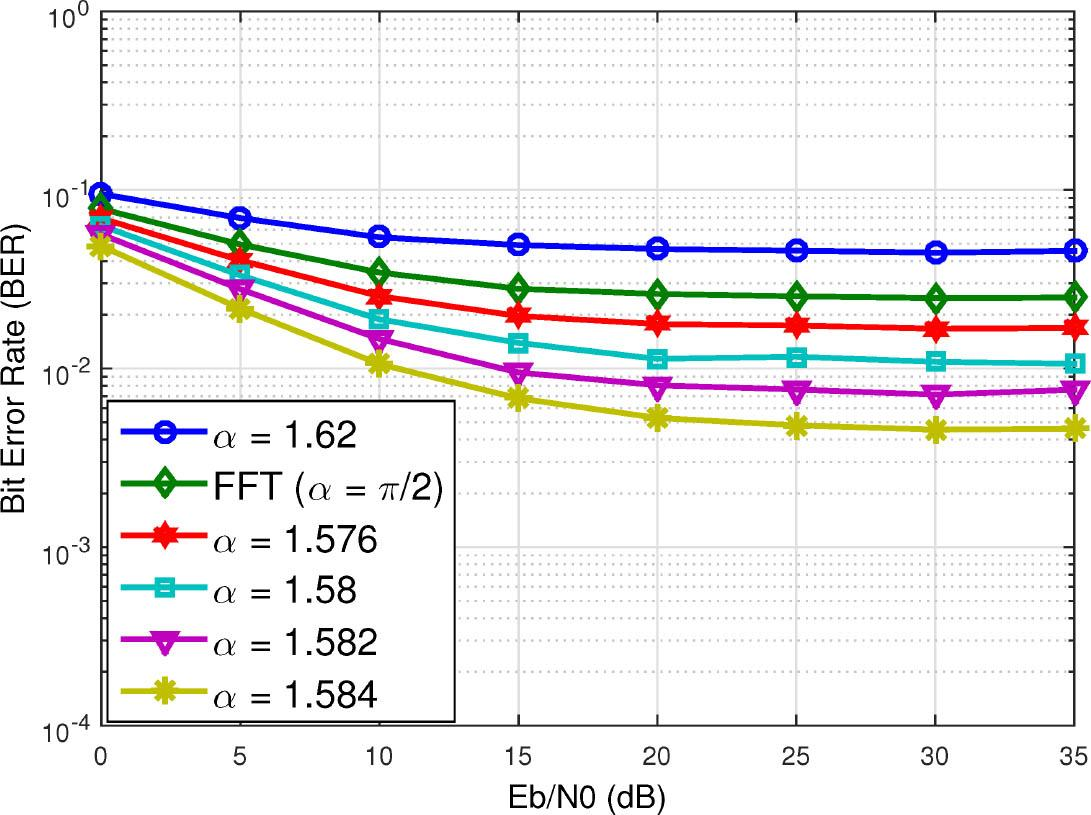FRFT and FT reduction PAPR comparison diagram based on SLM algorithm. FT, Fourier transform; FRFT, fractional Fourier transform; SLM, selective mapping.

It can be seen from Figure 2 that the PAPR of FRFT is further reduced based on FFT. When the threshold PAPR0 is around 6.6 dB, the probability that the PAPR under FRFT is higher than this threshold is <10−4, while the FFT result is >10−1. It can be seen that the probability of high PAPR is reduced by about 104, the highest PAPR under FRFT is about 6.6 dB, and the PAPR under FFT can reach 9 dB. For the sake of comparison, the time domain signals with a time of 0–6.243 8 μs and a power of −10 dBm–6 dBm are uniformly selected. The simulation results are shown in Figure 3 .

It can be seen from Figure 3 that the highest peak power of the original signal is around −6.05 dBm; after processing by the SLM algorithm, the highest peak power is around −6.27 dBm; and after processing by the FRFT-based SLM algorithm, the highest peak power is only −6.40 dBm. Moreover, the signal processed by the SLM algorithm based on FRFT has the least glitches and the smoothest signal. Therefore, by combining the FRFT algorithm, the PAPR suppression capability of SLM is further improved. In an actual system, one can consider sacrificing part of the PAPR suppression performance to improve the impact of the introduction of the SLM algorithm on the system. Since the introduction of the SLM algorithm will bring high complexity to the system, as the rotation factor increases, the complexity also doubles. The simulation results of the influence of the combination of FRFT and SLM algorithm on the complexity of the SLM algorithm are shown in Figure 4 .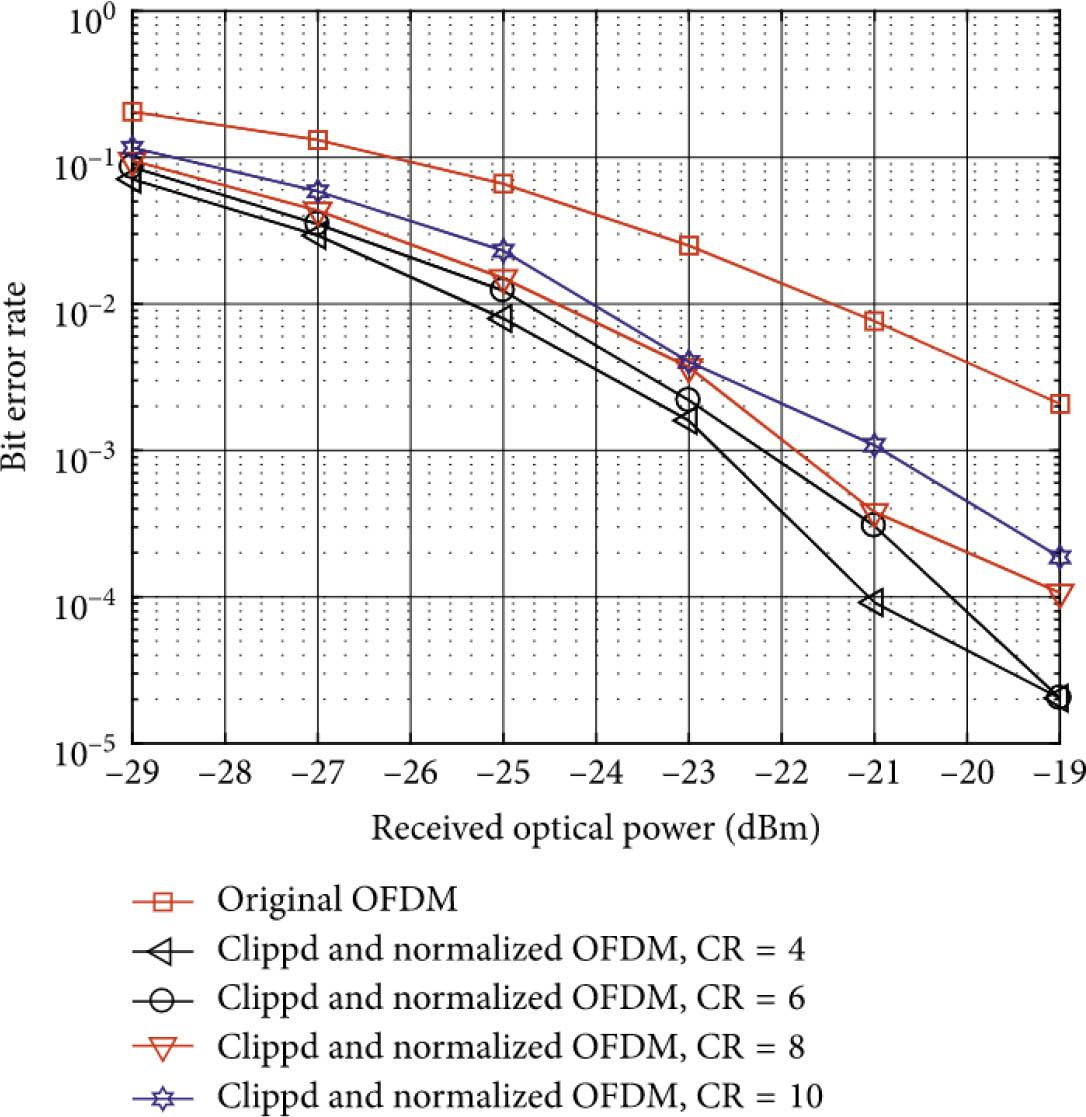Comparison of FRFT and FFT reduced PAPR with different twiddle factors under the SLM algorithm. FFT, fast Fourier transform; FRFT, fractional Fourier transform; SLM, selective mapping.

It can be seen from Figure 4 that the PAPR suppression ability when the number of twiddle factors D = 2 in the FRFT-based SLM algorithm lies between the suppression ability of D = 8 and D = 16 in the single SLM algorithm, and is equal to the suppression ability of D = 16. Closer. Therefore, using the SLM algorithm with D = 2 under FRFT to replace the SLM algorithm with D = 16 under FFT has similar PAPR suppression performance, but the introduction of the SLM algorithm reduces the complexity of the system by 8 times.

Limiting algorithm combined with FRFT

Limiting algorithms mainly achieve the purpose of suppressing PAPR through signal distortion. Here we consider the simplest peak clipping and limiting. First, the effectiveness of the combined algorithm is analysed. The limiting threshold in the limiting algorithm is 6.6, and the obtained CCDF curve is shown in Figure 5.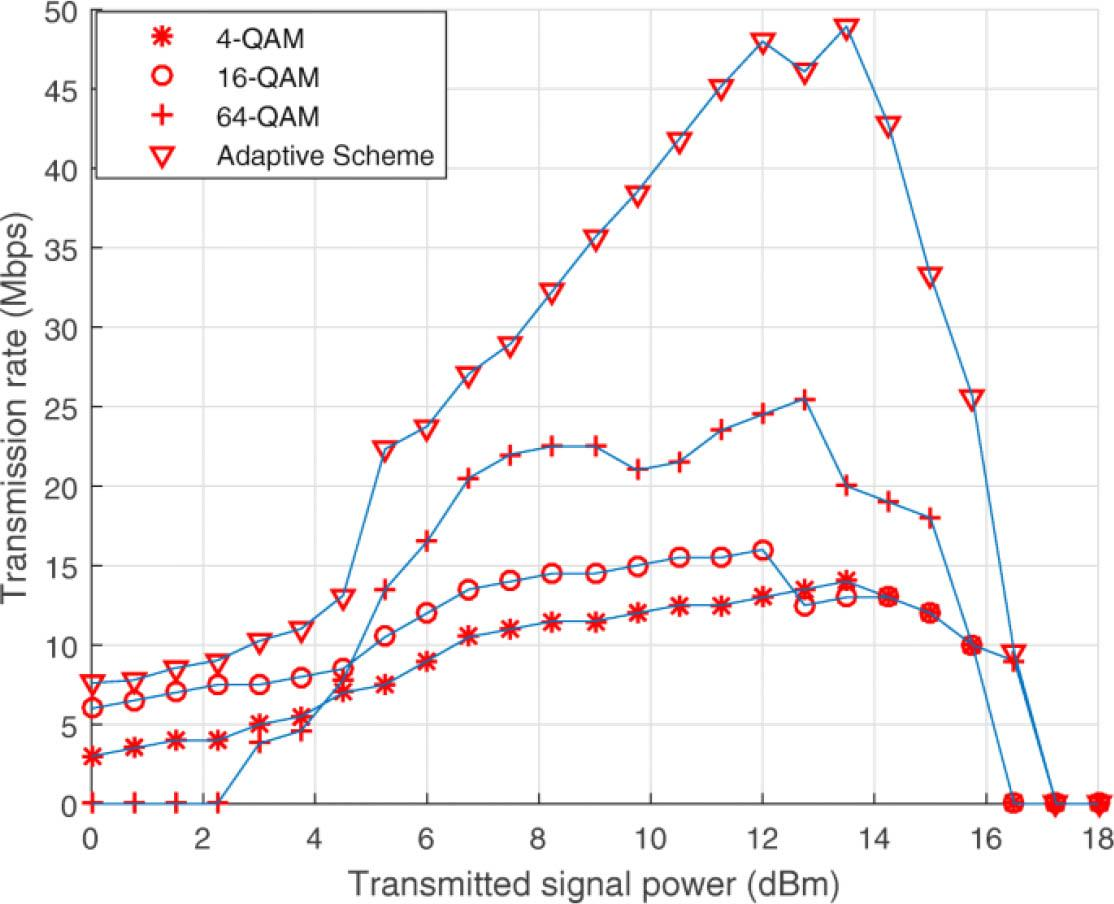FRFT and FFT reduce the PAPR comparison chart based on the limiting algorithm. FFT, fast Fourier transform; FRFT, fractional Fourier transform.

Due to the suppression characteristics of the limiting algorithm, the curve trends of the two processing signals are very similar. Compared with the SLM algorithm, the PAPR suppression effect of the limiting algorithm is more prominent. It can be seen from Figure 5 that the signal processed by FRFT is more sensitive to amplitude limiting. The probability that the PAPR of the signal processed by FRFT is >5.8 dB is 10−4, and the probability that the PAPR of the signal processed by FFT is >5.8 dB at this time Up to 0.8. The optical path simulation analysis of the combined algorithm is also performed, and the observation window is the same as the FRFT combined SLM algorithm. The simulation results are shown in Figure 6.

It can be seen from Figure 6 that the peak power of the original signal is −6.02 dBm; the peak power of the signal processed by the limiter is −6.30 dBm; the peak power of the signal processed by the FRFT-based limiter algorithm is −6.40 dBm. The FRFT combined with the limiting algorithm has the best glitch smoothness. Since the selected limit thresholds are the same, theoretically the highest PAPR values that can be reached by the two FT signals are the same. However, after 249,984 bit information is transmitted through the optical path, the peak value of the signal processed by FRFT is lower than the peak value of the signal processed by FFT. It can be seen that the combination of FRFT and limiting algorithm is more effective. The limiting algorithm will have an impact on the system's bit error rate performance. The two FTs combined with the limiting algorithm are simulated and analysed for the system's bit error rate performance after optical transmission. The simulation results are shown in Figure 7 .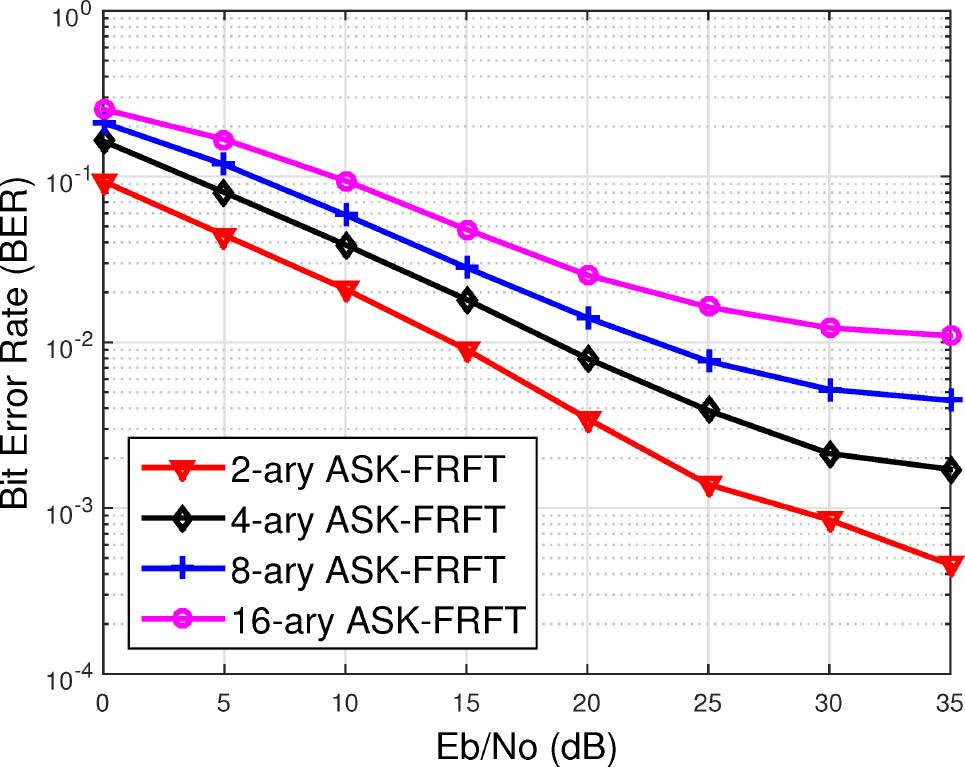Comparison of bit error rate between FRFT and FFT based on the limiting algorithm. FFT, fast Fourier transform; FRFT, fractional Fourier transform.

It can be seen from Figure 7 that the bit error rate performance of FRFT and FFT are equivalent, which conforms to the theoretical analysis. However, due to the introduction of the limiting algorithm, the bit error rate performance is significantly reduced. When the optical signal-to-noise ratio is 8 dB, the bit error rate increases from 10−4 to 10−3.

By increasing the limit threshold of the limit algorithm in the case of FRFT, the influence of the introduction of the limit algorithm on the bit error rate is improved. After many adjustments, when the limiting threshold is increased to 7.4, the FRFT combined with the limiting algorithm and the CCDF curve of the limiting algorithm when the threshold is 6.6 are basically the same, that is, the PAPR suppression performance of the two cases is basically the same. The results are as follows Figure 8. At this time, the bit error rate transmitted through the optical path is shown in Figure 9.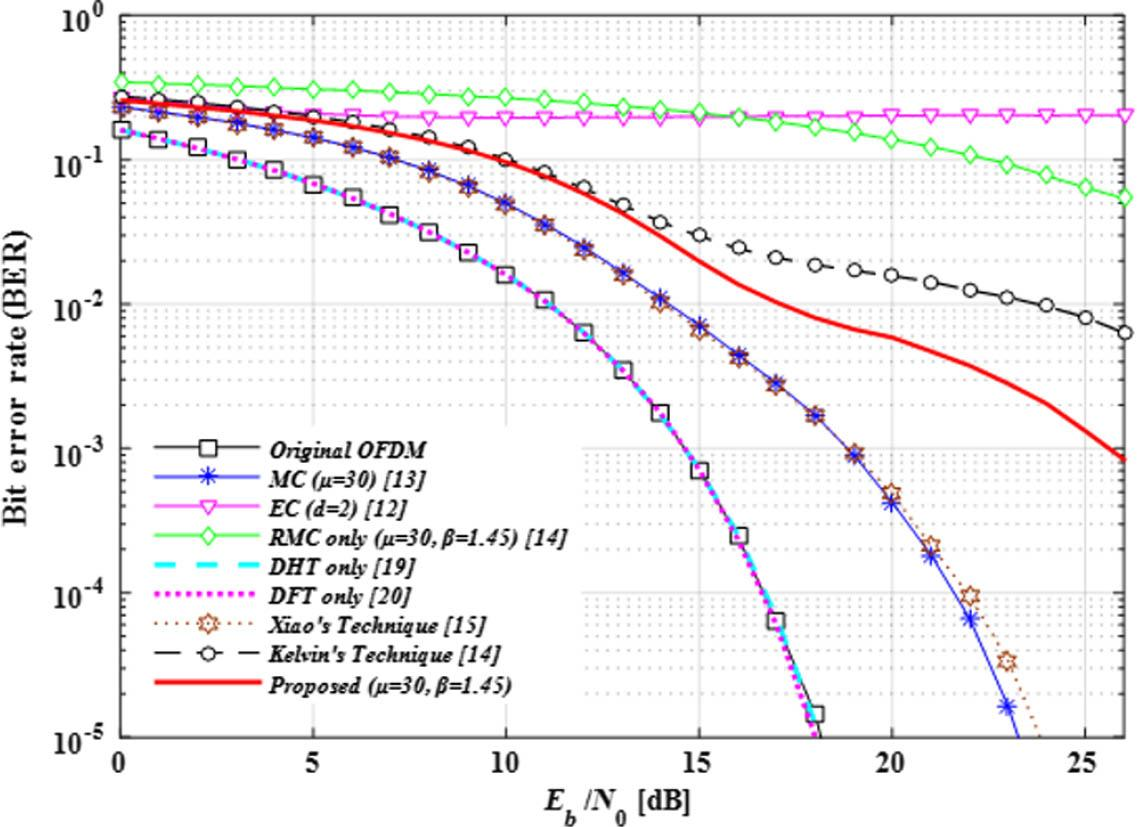Comparison of PAPR suppression with the original algorithm after raising the limit threshold in the case of FRFT. FRFT, fractional Fourier transform.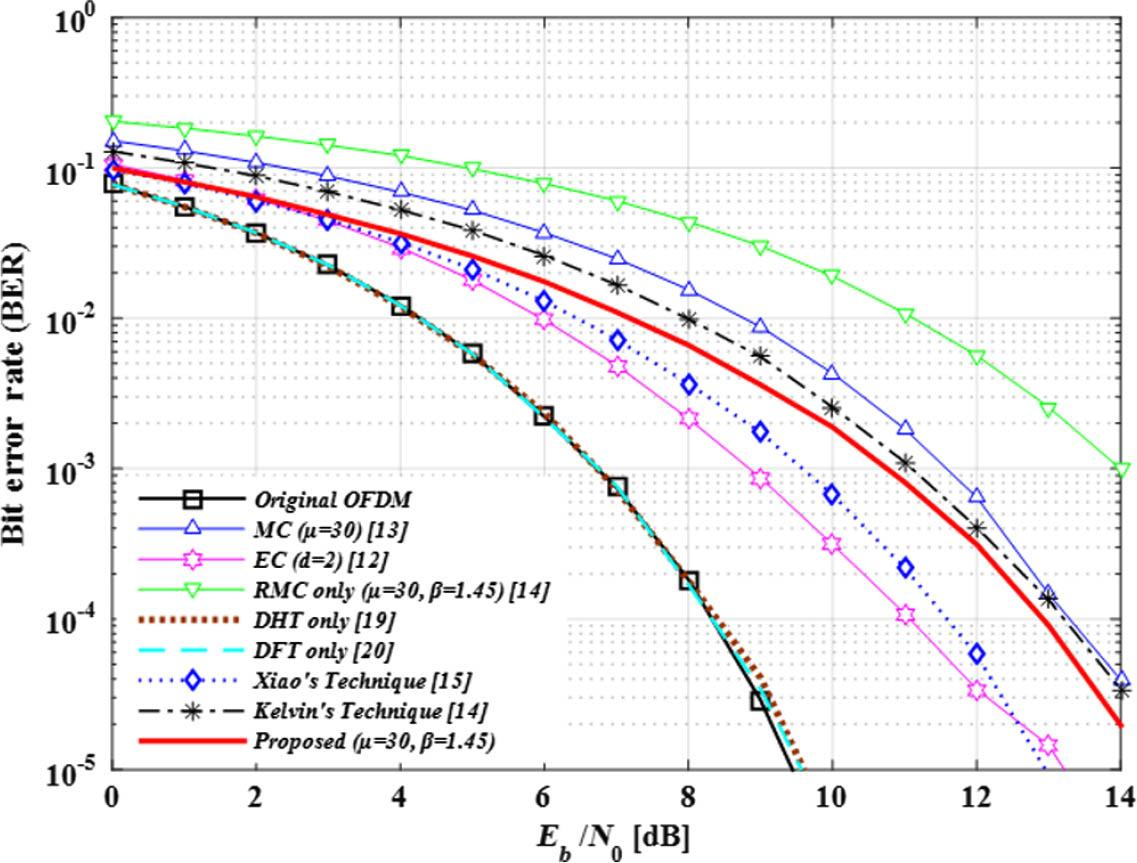Comparison of the bit error rate of the original algorithm PAPR after increasing the limit threshold in the case of FRFT. FRFT, fractional Fourier transform.

It can be seen from Figure 9 that after increasing the limiting threshold, the bit error rate performance of the signal processed by FRFT has been significantly improved. And as the optical signal-to-noise ratio increases, the improvement effect becomes more obvious. After the optical signal-to-noise ratio reaches 10 dB, the bit error rate of the signal processed by FRFT has been.

‘Ownership discrimination’ of financial repression

my country's financial repression has a clear state-owned bias. Financial institutions such as state-owned banks have long been in a monopoly position, and their capital rationing tends to favour state-owned economic departments and large enterprises. At the same time, the interest on loans received by state-owned economic departments is far below market levels. The World Bank's survey shows all the production scores of enterprises. The samples are divided according to whether they have a foreign capital component, whether a foreign capital component is >25%, and whether they have a state-owned component. Or equal to 25% are classified as non-foreign-funded enterprises, and the above sub-samples are grouped and regressed. The results are shown in Table 1. The explanatory variable is the proportion of informal financial financing. From the regression results, whether it is a foreign-funded enterprise or a foreign-funded enterprise, the company's informal financial financing ratio will not be affected by financial repression, and financial repression will not increase the enterprise's informal financial financing ratio. This may be due to the foreign capital component. The information asymmetry in the credit market is reduced. Formal financial institutions will think that enterprises with foreign-funded components have better credit qualifications. Therefore, they tend to lend to enterprises with foreign-funded components. This can alleviate the credit constraints of enterprises and avoid them. Will affect the proportion of informal financial financing of enterprises. The proportion of informal financial financing of companies that do not have foreign-invested components in the above sample is significantly affected by financial repression. At the same time, enterprises with state-owned components are not affected by financial repression in terms of informal financial financing. That is to say, a network of state-owned relationships can effectively alleviate the financing constraints of enterprises, and enterprises with state-owned components have closer relations with the government. It is easier to obtain the resources or credit needed for development from the government, so it has no significant impact on the proportion of informal finance, while financial repression has a significant positive effect on the proportion of informal finance for non-state-owned enterprises. The above results verify Hypothesis 1, that financial repression has significant characteristics of ‘discrimination on ownership’ .

Regression results of samples by ownership type.

Explained variable: proportion of informal financial financing Is there a foreign capital component Whether it is a foreign company Is there any state-owned content
fdi ≠ 0 fid = 0 fdi > 25% fdi ≤ 25% state > 0 state = 0

Financial repression 1.089 (0.75) 1.290 (3.56) 0.138 (0.10) 1.331 (3.66) 2.040 (0.89) 1.438 (3.51)
Enterprise size 0.144 (0.13) −0.103 (−0.39) 0.181 (0.15) −0.103 (−0.39) 0.122 (0.17) −0.045 (−0.19)
Enterprise growth rate 6.351 (2.06) 2.551 (2.81) 8.428 (2.29) 2.496 (2.78) −1.781 (−0.48) 3.202 (3.66)
Natural logarithm of business age 0.441 (0.39) −0.445 (−2.54) 0.098 (0.06) −0.437 (−2.56) 0.938 (0.99) −0.454 (−2.62)
Average years of education of employees −0.747 (−0.96) −0.650 (−4.24) −0.643 (−0.81) −0.651 (−4.27) −1.149 (−1.58) −0.674 (−4.91)
Constant term 5.055 (0.05) 47.791 (4.73) 34.407 (0.40) 47.177 (4.46) 86.840 (1.08) 39.579 (3.20)
N 124 1443 111 1451 74 1493
Adjusted R2 0.126 0.051 0.164 0.051 0.228 0.050
The ‘scale discrimination’ of financial repression

As far as the size of the enterprise is concerned, we guess that because small-scale enterprises have higher operating risks and fewer mortgageable assets, they are often discriminated against by financial institutions in the credit market, and the resources needed for development are more dependent on informal financial financing. The impact of financial repression is more serious; but when the degree of financial repression is high, the cost of formal financial financing rises and large enterprises will also prefer to choose informal financial financing. According to the grouping standard of the World Bank questionnaire, the number of employees in small companies is 5–19, the number of employees in medium-sized companies is 20–99, and the number of employees in large companies is 100 or more. The samples are divided according to this standard and the regression is performed. Analysis. We first use formal financial financing and informal financial financing as the explained variables to perform sub-sample regression. The regression results show that financial repression has significantly reduced the proportion of formal financial financing and increased the proportion of informal financial financing. There is no significant difference in different scales. We believe that this may be due to excessive financial repression, which has exceeded the critical value affecting state-owned enterprises and large enterprises. This is basically in line with our conjecture. Immediately after sub-sample regression with sub-indices of informal financial financing, it can be seen from Table 2 that the regression results basically verify Hypothesis 2 . In the sub-sample regression of enterprises of different sizes, the coefficients of financial repression are all positive, but the significance is different. In the case of deepening financial repression, companies will increase informal financial financing, while large companies will significantly increase commercial credit financing but have no significant impact on informal private lending and financing, and small companies will significantly increase informal private lending but have no significant impact on commercial credit financing. There was no significant impact. Medium-sized enterprises increased their commercial credit financing and informal private lending. This may be because large companies have more resources to establish relatively good business relationships with other companies, and therefore commercial credit financing will increase; while small companies cannot establish good business relationships with other companies due to differences in scale, so they only can rely on social networking and other informal private lendings. The regression results also confirm the large-scale discrimination of financial repression against commercial credit and informal private lending, as shown in Table 2 .

Regression results of samples divided by size.

Explained variable: Informal financial financing ratio index Commercial credit Informal private lending
Small Medium Large Small Medium Large

Financial repression 1.591 (1.03) 0.926 (1.44) 1.509 (3.67) 0.576 (1.68) 0.227 (1.02) 0.160 (1.15)
Percentage of foreign private, private and institutional shareholding −0.047 (−1.97) 0.022 (0.83) 0.015 (0.60) −0.001 (−0.12) −0.006 (−1.86) 0.005 (0.73)
The proportion of government shares −0.029 (−4.86) −0.022 (−4.37) −0.027 (−3.45) −0.010 (−1.48) −0.007 (−2.87) −0.004 (−1.97)
Enterprise size −0.072 (−0.10) 0.021 (0.08) −0.063 (−0.16) 0.042 (0.06) −0.080 (−1.02) −0.058 (−0.78)
Enterprise growth rate 4.302 (1.65) 2.246 (1.58) 1.836 (1.23) 0.828 (0.74) 0.514 (0.92) 0.521 (1.51)
Natural logarithm of business age −0.268 (−0.85) −0.643 (−2.67) 0.092 (0.65) −0.031 (−0.27) −0.218 (−2.12) −0.099 (−1.26)
Average years of education of employees −0.116 (−0.31) −0.148 (−0.60) −0.924 (−5.04) −0.248 (−0.89) −0.261 (−2.02) −0.181 (−2.03)
Constant term 84.816 (2.16) 41.169 (2.21) 13.055 (0.53) 7.382 (0.34) 19.201 (1.75) 11.921 (2.64)
N 207 642 718 207 639 717
R2 0.097 0.065 0.051 0.055 0.056 0.034
Conclusion

This paper analyses the limitations of the general cascade algorithm to the PAPR suppression performance of the OFDM system and the improvement of the system performance. On this basis, a more effective combination scheme based on FRFT is proposed. Combining the optical path simulation of MATLAB and Outstep, the effect of FRFT is analysed from the aspects of PAPR suppression performance and system performance improvement, and two types of algorithms are selected to investigate the universality of FRFT. The results show that FRFT has good results. From a more practical point of view, different functions of FRFT can be selected according to the adopted PAPR suppression algorithm and the specific requirements of the system. Using the SLM algorithm, it can be seen through simulation that the SLM algorithm has a weaker ability to suppress PAPR than the limiting algorithm, so it can be combined with the FRFT algorithm to further reduce the PAPR of the system. As for the limiting algorithm, because the algorithm itself has a strong PAPR suppression ability, but the algorithm has a great influence on the system's bit error rate performance, it can be combined with the FRFT algorithm to improve the system's bit error rate performance and achieve the purpose of reliable transmission. As a harmless algorithm, FRFT is a good choice when combined with the PAPR suppression algorithm.

#### Regression results of samples by ownership type.

Explained variable: proportion of informal financial financing Is there a foreign capital component Whether it is a foreign company Is there any state-owned content
fdi ≠ 0 fid = 0 fdi > 25% fdi ≤ 25% state > 0 state = 0

Financial repression 1.089 (0.75) 1.290 (3.56) 0.138 (0.10) 1.331 (3.66) 2.040 (0.89) 1.438 (3.51)
Enterprise size 0.144 (0.13) −0.103 (−0.39) 0.181 (0.15) −0.103 (−0.39) 0.122 (0.17) −0.045 (−0.19)
Enterprise growth rate 6.351 (2.06) 2.551 (2.81) 8.428 (2.29) 2.496 (2.78) −1.781 (−0.48) 3.202 (3.66)
Natural logarithm of business age 0.441 (0.39) −0.445 (−2.54) 0.098 (0.06) −0.437 (−2.56) 0.938 (0.99) −0.454 (−2.62)
Average years of education of employees −0.747 (−0.96) −0.650 (−4.24) −0.643 (−0.81) −0.651 (−4.27) −1.149 (−1.58) −0.674 (−4.91)
Constant term 5.055 (0.05) 47.791 (4.73) 34.407 (0.40) 47.177 (4.46) 86.840 (1.08) 39.579 (3.20)
N 124 1443 111 1451 74 1493
Adjusted R2 0.126 0.051 0.164 0.051 0.228 0.050

#### Regression results of samples divided by size.

Explained variable: Informal financial financing ratio index Commercial credit Informal private lending
Small Medium Large Small Medium Large

Financial repression 1.591 (1.03) 0.926 (1.44) 1.509 (3.67) 0.576 (1.68) 0.227 (1.02) 0.160 (1.15)
Percentage of foreign private, private and institutional shareholding −0.047 (−1.97) 0.022 (0.83) 0.015 (0.60) −0.001 (−0.12) −0.006 (−1.86) 0.005 (0.73)
The proportion of government shares −0.029 (−4.86) −0.022 (−4.37) −0.027 (−3.45) −0.010 (−1.48) −0.007 (−2.87) −0.004 (−1.97)
Enterprise size −0.072 (−0.10) 0.021 (0.08) −0.063 (−0.16) 0.042 (0.06) −0.080 (−1.02) −0.058 (−0.78)
Enterprise growth rate 4.302 (1.65) 2.246 (1.58) 1.836 (1.23) 0.828 (0.74) 0.514 (0.92) 0.521 (1.51)
Natural logarithm of business age −0.268 (−0.85) −0.643 (−2.67) 0.092 (0.65) −0.031 (−0.27) −0.218 (−2.12) −0.099 (−1.26)
Average years of education of employees −0.116 (−0.31) −0.148 (−0.60) −0.924 (−5.04) −0.248 (−0.89) −0.261 (−2.02) −0.181 (−2.03)
Constant term 84.816 (2.16) 41.169 (2.21) 13.055 (0.53) 7.382 (0.34) 19.201 (1.75) 11.921 (2.64)
N 207 642 718 207 639 717
R2 0.097 0.065 0.051 0.055 0.056 0.034

Dusunceli F. New Exact Solutions for Generalized (3+1) Shallow Water-Like (SWL) Equation[J]. Applied Mathematics and Nonlinear Sciences, 2019, 4(2):365–370. DusunceliF New Exact Solutions for Generalized (3+1) Shallow Water-Like (SWL) Equation[J] Applied Mathematics and Nonlinear Sciences 2019 4 2 365 370 10.2478/AMNS.2019.2.00031 Search in Google Scholar

Zhang Y, Li H, Clark J D. Experimental simulation of mathematical learning process based on ’chunk-objective’[J]. Applied Mathematics and Nonlinear Sciences, 2020, 5(2):425–434. ZhangY LiH ClarkJ D Experimental simulation of mathematical learning process based on ’chunk-objective’[J] Applied Mathematics and Nonlinear Sciences 2020 5 2 425 434 10.2478/amns.2020.2.00061 Search in Google Scholar

Nystrom H E, Watkins S E, Nanni A, et al. Financial Viability of Fiber-Reinforced Polymer (FRP) Bridges[J]. Journal of Management in Engineering, 2015, 19(1):2–8. NystromH E WatkinsS E NanniA Financial Viability of Fiber-Reinforced Polymer (FRP) Bridges[J] Journal of Management in Engineering 2015 19 1 2 8 10.1061/(ASCE)0742-597X(2003)19:1(2) Search in Google Scholar

B J C G A, C R C M Y. Effects of government financial incentives on firms’ innovation performance in China: Evidences from Beijing in the 1990s[J]. Research Policy, 2015, 44(1):273–282. B J C G A, C R C M Y Effects of government financial incentives on firms’ innovation performance in China: Evidences from Beijing in the 1990s[J] Research Policy 2015 44 1 273 282 10.1016/j.respol.2014.09.001 Search in Google Scholar

Baker S. Paper F2 Financial Management: When It Comes to Tackling the Written Section of the Analysis and Interpretation Question, Too Many F2 Candidates Deny Themselves Easy Marks by Ignoring or Forgetting the Clear Prompts Provided[J]. Physica Scripta, 2015, 90(2):981–984. BakerS Paper F2 Financial Management: When It Comes to Tackling the Written Section of the Analysis and Interpretation Question, Too Many F2 Candidates Deny Themselves Easy Marks by Ignoring or Forgetting the Clear Prompts Provided[J] Physica Scripta 2015 90 2 981 984 Search in Google Scholar

Frydman C, CF Camerer. The Psychology and Neuroscience of Financial Decision Making[J]. Trends in Cognitive Sciences, 2016, 20(9):661–675. FrydmanC CamererCF The Psychology and Neuroscience of Financial Decision Making[J] Trends in Cognitive Sciences 2016 20 9 661 675 10.1016/j.tics.2016.07.003 Search in Google Scholar

Riosperez J A, Rai S, Miller D M. Differences in financial disparities in Kentucky compared to the U.S. national level.[J]. Macromolecules, 2015, 37(16):5860–5862. RiosperezJ A RaiS MillerD M Differences in financial disparities in Kentucky compared to the U.S. national level.[J] Macromolecules 2015 37 16 5860 5862 Search in Google Scholar

Jain S H, Powers B W, Hawkins J B, et al. The digital phenotype[J]. Nature Biotechnology, 2015, 33(5):462–3. JainS H PowersB W HawkinsJ B The digital phenotype[J] Nature Biotechnology 2015 33 5 462 3 10.1038/nbt.3223 Search in Google Scholar

Yue D, Kong Y, Feng B, et al. Sparse Coding Inspired Optimal Trading System for HFT Industry[J]. IEEE Transactions on Industrial Informatics, 2015, 11(2):1–1. YueD KongY FengB Sparse Coding Inspired Optimal Trading System for HFT Industry[J] IEEE Transactions on Industrial Informatics 2015 11 2 1 1 Search in Google Scholar

Nazlioglu S, Soytas U, Gupta R. Oil prices and financial stress: A volatility spillover analysis[J]. Energy Policy, 2015, 82(jul.):278–288. NazliogluS SoytasU GuptaR Oil prices and financial stress: A volatility spillover analysis[J] Energy Policy 2015 82 jul. 278 288 10.1016/j.enpol.2015.01.003 Search in Google Scholar

• #### Fractional Linear Regression Equation in Agricultural Disaster Assessment Model Based on Geographic Information System Analysis Technology

Recommended articles from Trend MD